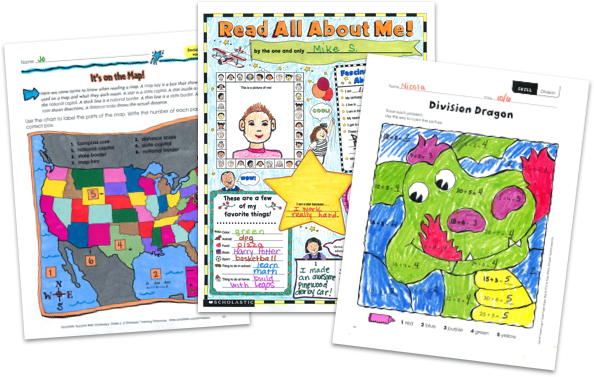MY FILE CABINET# Algebra Worksheets for Basic & Advanced LevelsScholastic Teachables (formerly Scholastic Printables) has hundreds of worksheets, games, and activities to help your students master the fundamentals of algebra. Make learning fun with engaging supplemental materials that cover everything from basic expressions to complex equations!

Scholastic Teachables (formerly Scholastic Printables) has hundreds of worksheets, games, and activities to help your students master the fundamentals of algebra. Make learning fun with engaging supplemental materials that cover everything from basic expressions to complex equations!

## Scholastic's Algebra Printables Cover Many Skills:

• Algebraic Expressions
• Equations
• Functions Formulas
• Integers
• Multiplication and Division
• Graphs & Tables
• Ordered Pairs
• Problem Solving
• Order of Operations
• Word Problems
• Logical Reasoning
• Prime Factors
• Greatest Common Factor
• Least Common Multiple Exponents
• Square Roots
• Mean, Median, Mode
• Representation
• Reasoning and Proofs
• Data Analysis
• Probability
• Properties Measurement
• Mathematical Models
• Ratios and Proportions
• Simplifying Expressions

## Sample Algebra Worksheets

Hundreds of Scholastic printables cover algebra and algebra readiness skills for students in K through grade 8! Sign up and you'll also get access to Scholastic's more than 30,000 award-winning printables and leveled learning packs in reading and math to reach every learner.

New printables and algebra worksheets are published each month to keep your courses fresh, exciting and up-to-date, and you can access all of our resources from any device, whether at school or at home. Spend more time focusing on your courses and less time on preparing materials—simply find it, print it, teach it!

Here's a sample of algebra worksheets for you to try in your class—FREE with a 30-day trial or subscription.

## Scholastic's Algebra Printables Cover Many Skills:

• Algebraic Expressions
• Equations
• Functions Formulas
• Integers
• Multiplication and Division
• Graphs & Tables
• Ordered Pairs
• Problem Solving
• Order of Operations
• Word Problems
• Logical Reasoning
• Prime Factors
• Greatest Common Factor
• Least Common Multiple Exponents
• Square Roots
• Mean, Median, Mode
• Representation
• Reasoning and Proofs
• Data Analysis
Probability
• Properties Measurement
• Mathematical Models
• Ratios and Proportions
• Simplifying Expressions
• Algebraic Expressions
• Equations
• Functions Formulas
• Integers
• Multiplication and Division
• Graphs & Tables
• Ordered Pairs
• Problem Solving
• Order of Operations
• Word Problems
• Logical Reasoning
• Prime Factors
• Greatest Common Factor
• Least Common Multiple Exponents
• Square Roots
• Mean, Median, Mode
• Representation
• Reasoning and Proofs
• Data Analysis
• Probability
• Properties Measurement
• Mathematical Models
• Ratios and Proportions
• Simplifying Expressions

## Sample Algebra Worksheets

Hundreds of Scholastic printables cover algebra and algebra readiness skills for students in K through grade 8! Sign up and you'll also get access to Scholastic's more than 30,000 award-winning printables and leveled learning packs in reading and math to reach every learner.

New printables and algebra worksheets are published each month to keep your courses fresh, exciting and up-to-date, and you can access all of our resources from any device, whether at school or at home. Spend more time focusing on your courses and less time on preparing materials—simply find it, print it, teach it!

Here's a sample of algebra worksheets for you to try in your class—FREE with a 30-day trial or subscription.## Additional Algebra Worksheets (Subscription Required)

Try FREE for 30 days!

If you are not 100% satisfied cancel during the FREE trial and owe nothing. If you are not 100% satisfied cancel during the FREE trial and owe nothing.